Mobile QR Code1. (Department of Aerospace Control Systems, Le Quy Don Technical University, Hanoi, Vietnam {nguyenngochungtk40, caohuutinhmta, langphong, phamngocbmtl}@gmail.com )

Object tracking, Thermal imaging sequences, Image processing, Real-time systems, MPSoC

## 1. Introduction

Object tracking algorithms for image processing have been widely researched. Algorithms perform object recognition using many characteristics, such as color, gray histogram, geometrical features, image intensity, and optical flow. From the properties of the object, a previous study  provided three widely used approaches for object tracking. The first approach is point tracking, in that objects are presented by their feature points. Some algorithms, such as Kalman Filter and Particle Filter, use this approach. The second is to track the object based on its centroid, e.g., Meanshift and Camshift. The third approach is shape-based tracking. Currently, this method has attracted considerable attention because of the development of artificial intelligence, which allows object recognition and tracking with high accuracy . On the other hand, these algorithms are usually too complex to run in real-time and require the characteristics of the target in advance.

The purpose of this research was to develop a real-time algorithm for tracking moving objects in a thermal imaging camera. The thermal objects considered in this paper have some characteristics, such as at a far distance from the camera, their sizes in the thermal image are quite small (only taking up a few pixels to tens of pixels). Typical examples of objects include airplanes and helicopters, which are described elsewhere . Therefore, the algorithm must work under the following conditions: the objects may be temporarily obscured; other thermal objects may be moving in the image simultaneously; the image of the tracked object can have a low resolution with no clear geometrical features.

This study does not use artificial intelligence to improve the quality of tracking moving objects in thermal images in the above conditions. Instead, it examines the possibility of combining algorithms using the first and second approaches with the analysis below.

Because algorithms using the first and second approaches are simple, they are suitable for computationally limited tracking systems. On the other hand, these algorithms often fail to track the correct object with transient occlusion and are sensitive to thermal noise. Combining algorithms can preserve their advantages and overcome their disadvantages, improving tracking system quality.

Combining the Camshift tracking algorithm with the Differential Frame detection algorithm was proposed . On the other hand, it does not fix the target-masking problem. Another study  successfully used the Kalman filter to deal with transitory target loss concerns. Nevertheless, it still required better target detection to avoid being followed by the wrong object. Another way of combining algorithms is described elsewhere , in which multiple methods were used to track objects and combine the results. This strategy made it difficult to take advantage of each algorithm.

This paper proposes a novel method of combining algorithms. Image intensity filters according to the speed and direction of motion are proposed to distinguish objects from noise or other moving objects better. After being processed by the threshold cutter, the input thermal image, will be filtered according to the difference between the optical flow information [7,8] and the velocity vector of the object being tracked. As a result, the intensity of the tracked object will be highlighted, while other moving objects will be blurred. The Camshift  algorithm can then be used to find the new centroid and size of the tracked object. The new position and size will be the input for the Kalman filter . The estimated information about the coordinates of the object on the image, including position, size, and velocity, will be used for the next timestep.

The experiment revealed positive tracking results from the proposed algorithm with a high processing speed. The algorithm also works well when the objects are temporarily obscured or when there are flares in the thermal images.

The algorithm was implemented using MPSoC technology to ensure the practicality of the new tracking algorithm on compact embedded devices. This combines FPGA and embedded system technology, allowing for high-speed computations and flexibility in performing the algorithms by embedding software on processors or by FPGA hardware.

The remainder of the article presents the new algorithm for thermal object tracking and experimental results and evaluation.

## 2. Proposed Fusion of Object Tracking Algorithms

The article proposes a new approach that combines detections, tracking algorithms, and the Kalman filter, as shown in Fig. 1. This is a real-time approach that effectively tracks the object in thermal image sequences, eliminates noise, and automatically re-tracks the object after being temporarily lost.

After initialization of system parameters and selection of tracked objects, the process of object tracking is performed in the following order:

##### Fig. 1. Structure of the new object tracking system.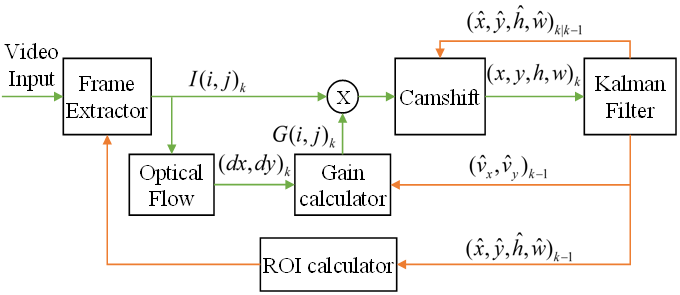· The Region of Interest (ROI), an area in which the object is being searched for, is extracted from the thermal image received from the video input to reduce the computation for the next algorithms and improve the ability of the system to respond in real-time. The ROI depends on estimated position and size states from the previous timestep ($\textit{k-1}$) of the Kalman filter $(\hat{x},\hat{y},\hat{h},\hat{w})_{k-1}$.

· The intensity of each pixel $(i,j)$in the ROI will be then filtered by the current gain $G(i,j)_{k}\,.$ $G(i,j)_{k}$ depends on the difference between the velocity vector of the object $(\hat{v}_{x},\hat{v}_{y})_{k-1}$ at the previous timestep and the Optical Flow vector $(dx,dy)_{k}$at the current timestep$\textit{.}$ As a result, the intensity of other moving objects is reduced. Therefore, the new system can decrease the possibility of tracking the wrong object. At the first timestep, the Gain and ROI values are initialized with a constant.

· The new position $(x,y)_{k}$ and size $(h,w)_{k}$of the tracked object in the image will be calculated using the Camshift algorithm. The inputs of this algorithm are the image at the current timestep and the predicted position and size of the tracked object from the Kalman filter at the previous timestep. $(\hat{x},\hat{y},\hat{h},\hat{w})_{k|k-1}$.

· Finally, the Kalman filter algorithm will estimate the location, size, and velocity of the tracked object through two steps: prediction and update. In the update step, outputs $(x,y)_{k}$ and $(h,w)_{k}$ from Camshift are used only when the tracked object is detected by the threshold detector in the region of interest. This is because the estimated velocity $(\hat{v}_{x},\hat{v}_{y})_{k}$ of the tracked object will be used to calculate the gain, the estimated position, and size $(\hat{x},\hat{y},\hat{h},\hat{w})_{k}$ will be used to calculate the region of interest at the next timestep. The prediction step will calculate the new position and size information $(\hat{x},\hat{y},\hat{h},\hat{w})_{k+1|k}$ of the object to be used as inputs for the Camshift algorithm at the next timestep.

The new proposed solution combines the Kalman, Camshift, and Optical Flow tracking algorithms, so this method is called the KCOF (Kalman-Camshift-Optical Flow). The details about the algorithms in Fig. 1 are given below.

### 2.1 Optical Flow Estimation

Optical Flow algorithms have been studied widely to identify moving objects. Lucas-Kanade , Farneback Optical Flow , and TV-L1 Optical Flow  are all well-known algorithms. Lucas-Kanade is simple but is not very accurate. TV-L1 is very accurate but complicated and requires more calculations. The Farneback Optical Flow algorithm has average complexity and accuracy. Optical Flow evaluation algorithms often use a pyramid model with many layers from coarse to fine to accurately determine the Optical Flow and reduce the number of calculations. The Farneback Optical Flow algorithm was chosen due to the real-time requirement. Gunnar Farneback proposed this algorithm. The idea is to model the object as a polynomial function, then find the motion sign between two images based on the expansion of the polynomials. Farneback used polynomials with eight parameters for 2D models to find the Optical Flow; more details are presented elsewhere .

### 2.2 Camshift Algorithm

The Camshift algorithm was developed from the Meanshift algorithm by adding the ability to detect changes in the object size during tracking. This is an algorithm that tracks the center of the color probability distribution of the object. In the case of thermal images, it tracks the center of the intensity of the object. Hence, this algorithm is suitable for tracking thermal objects at long distances when their images are unclear. The Camshift algorithm is computationally simple and can be run in real-time. On the other hand, it often fails when there are multiple objects with similar intensities in the image. The detail of this algorithm can be found elsewhere [9,10]. The outputs of the Camshift algorithm are the centroid position of the search window and the size of the tracked object. They will be the measurement states of the Kalman filter.

### 2.3 Kalman Filter

The moving object is presented by state variables, including the position $(x,y)$, velocity $(v_{x},v_{y})$, size $(h,w)$, and change speed of size $(v_{h},v_{w})$:

##### (1)
$\mathbf{x}=\left[\begin{array}{llllllll} x & v_{x} & y & v_{y} & h & v_{h} & w & v_{w} \end{array}\right]$

The dynamic linear model:

$\begin{array}{l} \mathbf{x}_{k}=F\,\mathbf{x}_{k-1}+\mathbf{w}_{k}\\ \mathbf{z}_{k}=H\,\mathbf{x}_{k}+\mathbf{\upsilon }_{k} \end{array}$

where

##### (2)
$F=\left[\begin{array}{llllllll} 1 & T & 0 & 0 & 0 & 0 & 0 & 0\\ 0 & 1 & 0 & 0 & 0 & 0 & 0 & 0\\ 0 & 0 & 1 & T & 0 & 0 & 0 & 0\\ 0 & 0 & 0 & 1 & 0 & 0 & 0 & 0\\ 0 & 0 & 0 & 0 & 1 & T & 0 & 0\\ 0 & 0 & 0 & 0 & 0 & 1 & 0 & 0\\ 0 & 0 & 0 & 0 & 0 & 0 & 1 & T\\ 0 & 0 & 0 & 0 & 0 & 0 & 0 & 1 \end{array}\right],\\ H=\left[\begin{array}{llllllll} 1 & 0 & 0 & 0 & 0 & 0 & 0 & 0\\ 0 & 0 & 0 & 0 & 0 & 0 & 0 & 0\\ 0 & 0 & 1 & 0 & 0 & 0 & 0 & 0\\ 0 & 0 & 0 & 0 & 0 & 0 & 0 & 0\\ 0 & 0 & 0 & 0 & 1 & 0 & 0 & 0\\ 0 & 0 & 0 & 0 & 0 & 0 & 0 & 0\\ 0 & 0 & 0 & 0 & 0 & 0 & 1 & 0\\ 0 & 0 & 0 & 0 & 0 & 0 & 0 & 0 \end{array}\right]$

where $\textit{T}$ is a sampling period; $\mathbf{w}_{k}$ and $\mathbf{\upsilon }_{k}$ are the process noise and measurement noise, respectively. The Kalman filter is performed in two steps.

Predict step:

##### (3)
$\begin{array}{l} \hat{\mathbf{x}}_{k|k-1}=F\,\hat{\mathbf{x}}_{k-1}\\ \mathbf{P}_{k|k-1}=F\,\mathbf{P}_{k-1}F^{T}+\mathbf{Q} \end{array}$

Measurement Update step:

##### (4)
$\begin{array}{l} \mathbf{K}_{k}=\mathbf{P}_{k|k-1}\mathbf{H}^{T}\left(\mathbf{H}\,\mathbf{P}_{k|k-1}\mathbf{H}^{T}+\mathbf{R}\right)^{-1}\\ \hat{\mathbf{x}}_{k}=\hat{\mathbf{x}}_{k|k-1}+\mathbf{K}_{k}\left(\mathbf{z}_{k}-\mathbf{H}\,\hat{\mathbf{x}}_{k|k-1}\right)\\ \mathbf{P}_{k}=\left(\mathbf{I}-\mathbf{K}_{k}\mathbf{H}\right)\mathbf{P}_{k|k-1} \end{array}$

After applying the Kalman filter, the ROI is calculated based on the estimated values. This improves tracking stability significantly and removes temporary occlusions and noise.

### 2.4 Gain Calculator

The gain value $G\left(i\,,j\right)\in [0,1]$ is determined by (5), which depends on the difference between the normalized velocity vector of the tracked object $\left(\hat{v}_{x}\,,\hat{v}_{y}\right)\in [-0.5,0.5]$ and the normalized Optical Flow vector $\left(dx\,,dy\right)\in [-0.5,0.5]$ at point $\left(i\,,j\right)$:

##### (5)
$G\left(i,j\right)=1-\left| dx-\hat{v}_{x}\right| \times \left| dy-\hat{v}_{y}\right|$

$G\left(i\,,j\right)$ will be maximum when the velocity vector and the Optical Flow vector match each other.

### 2.5 Analysis of the Proposed Method

The Camshift algorithm is used to measure the new size and position of the tracked object (Fig. 1). The results of these measurements are inputs to the Kalman filter. The Camshift algorithm was chosen because of its suitability for tracking the desired objects. It has the well-adapted ability to change the shape and size of the tracked object and perform a simple computation. On the other hand, the Camshift algorithm cannot detect an occluded object. Therefore, the Kalman filter is needed to remember the target being tracked. Compared to the Kalman filter reported elsewhere [9,10], more states of the object are estimated, including size and derivative of size.

The Camshift algorithm can fail and track the wrong object when some objects have the same intensity as the tracked object. To solve this problem, this paper proposed an intensity filter in which the gain of the filter depends on the velocity of the tracked object and others. The velocity of the tracked object is estimated using the Kalman filter, while the velocities of others are obtained by the Optical Flow algorithm. The gain of the filter is derived from (5). As a result, objects with movements different from the tracked object will be reduced in intensity. Therefore, the Camshift algorithm can avoid tracking the wrong object.

## 3. Experimental Setting

Experiments were performed using thermal imaging sequences collected from the PICO640-GEN2 sensor produced by ULIS (France) to verify the effectiveness of the proposed fusion algorithm. The image preprocessing algorithms are listed elsewhere . Thermal imaging sequences have some parameters: 640${\times}$480 resolution, 60 FPS, and LWIR (8-14 ${\mathrm{\mu}}$m).

The tracking algorithms were processed on two different devices for comparison. The first device was a PC using CPU Core i7-6820HQ @ 2.70GHz, RAM 16GB, GPU Quadro M1000M. The second was an MPSoC UltraScale+ ZU4EV-1E including CPU Quad-Core ARM Cortex-A53 1.3GHz, Dual-core ARM Cortex-R5 533MHz, Logic Cells 192K, and 2GB DDR4 SDRAM.

The PICO640-GEN2 sensor was connected to the PC via USB 3.0, while it was connected to the MPSoC via a native video interface. The video stream received from the sensor was converted to the interface type in the Video Input block to be compatible with the input of VDMA (Video Direct Memory Access). The thermal imaging data were recorded in the DDR memory. This helps reduce the CPU work and improves the run-time computing of the image processing. Fig. 2 presents the structure of the processing system.

The implementation of the thermal image object tracking system on MPSoC in Fig. 2 consisted of two main parts: the first was built on a programmable logic block (PL) that is responsible for collecting thermal image data from the sensor, preprocessing, saving the image to DRAM, executing the tracking algorithm, and displaying the data. The second part was the microprocessor block that maintains the operation of the PL side function blocks, exchanges computational parameters, and controls the storage of data in memory. Information exchange between PS and PL was done using the AXI bus (Advanced eXtensible Interface). The AXI4 Lite bus was used to configure, control, and exchange individual information from the CPU to the IP cores in the PL block. The AXI Stream bus was used to write and read image sequences between the DDRAM and function blocks.

##### Fig. 2. Structure of the new object tracking system implemented in MPSoC.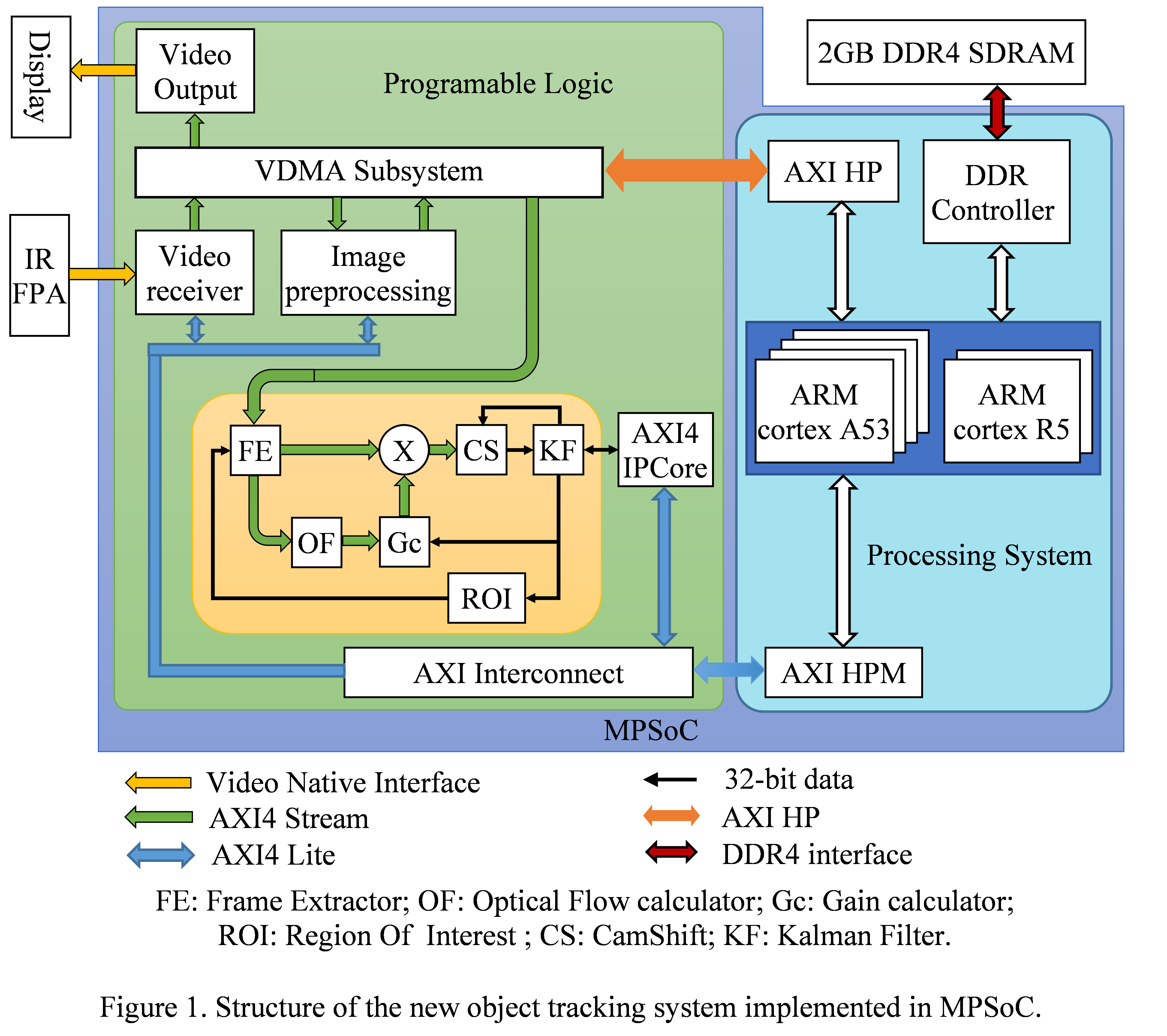Data from the thermal sensor were collected using the Video Receiver block, which was built on the VHDL language and the IP cores of Xilinx. First, the video was converted to the AXI4 Stream format, and the data was written into DDRAM by the VDMA block. Second, to display the image data, the data from DDRAM was read using the VDMA block, and the Video Output block will convert the data from AXI4 Stream to the video interface standard for the display. The address of the data written and read on DDRAM was controlled by the program in the CPU.

The image preprocessing and object tracking algorithms were all written in C$^{++}$ language and compiled into kernels for acceleration in the PL blocks using the Vitis HLS synthesis tool. This allows the above algorithms to be developed and tested first in C$^{++}$. Therefore, it helps reduce the development time and increase the stability of the algorithms.

The image data from DDRAM is transmitted to the input of the tracking block by Data Mover built by VDMA IP. The tracking block consists of kernels (FE, OF, ROI, CS, KF, and Multiplier), which are compiled from C$^{++}$ language to hardware IP in the PL. These kernels execute the functional algorithms, exchanging data with global memory in DDRAM. The kernels can also use streaming connections to communicate with others. The host program running on the CPU can set up input parameters and trigger the execution of kernels. Finally, the output data of the tracking algorithm will be passed to the host program by the AXI4 IPCore.

The advantage of implementing kernels in PL is the high computational speed. There are many iterations for each operator because kernels often have to calculate for each pixel. The solution to this problem is to use parallel computation on hardware, accelerating computation many times over performing kernels in the CPU. On the other hand, the balance between using the number of parallel operations and the computation time is important to ensure the real-time requirements of the object-tracking algorithm due to the resource limitations of MPSoC.

## 4. Results and Evaluation

To evaluate the efficiency of the newly proposed fusion algorithm and compare it with other object tracking algorithms, the experiments in this paper were conducted in three different circumstances: the first circumstance - changes in the size of the tracked object; the second circumstance - temporarily obscured object; the third circumstance - there is an active thermal noise. The algorithms used for comparison were algorithms that guarantee high real-time, such as MIL , TLD , KCF , MedianFlow , CSRT , MOSSE , and BOOSTING . Thermal imaging sequences were collected in the laboratory using the PICO640-GEN2 sensor. They have a 640${\times}$480 resolution and 60 FPS. Thermal objects were produced in many different circumstances. They simulated some real objects that need to be tracked.

Fig. 3 presents four frames extracted from the video. The proposed new algorithm can track the object well when its size and direction change quickly. While the TLD and MedianFlow algorithms are not adapted to the change of object size, the MIL, KCF, CSRT, MOSSE, and BOOSTING algorithms cannot track the object when moving and changing direction at high velocity.

First circumstance - changes in the size of the tracked object:

##### Fig. 3. Results of the experiment of tracking algorithms when resizing.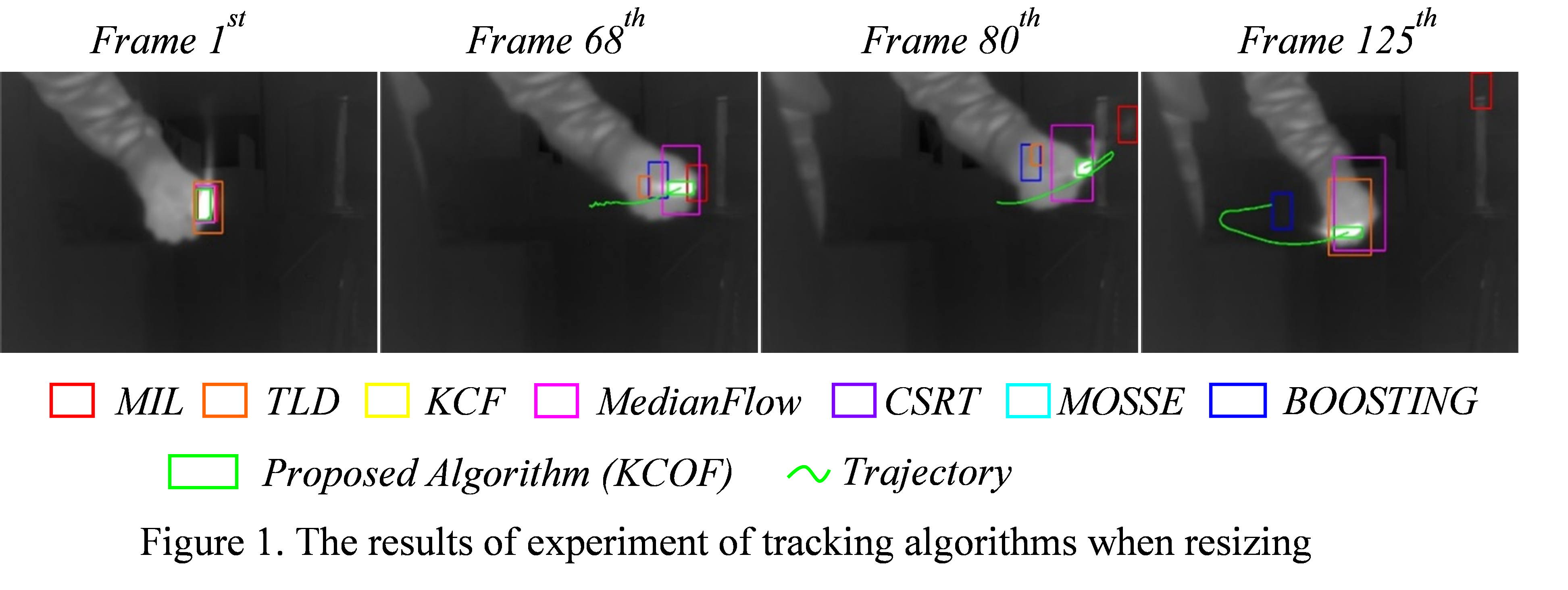The change in size and shape of the tracked object significantly affects the performance of the feature-based or the shape-based tracking algorithms, such as KCF, CSRT, and MOSSE. These tracking algorithms need to update the database or the template because the change in shape and size leads to a major modification in the features of the tracked object. Furthermore, the change speed is fast. Therefore, they are easy to fail. In this case, the TLD and MedianFlow algorithms can still track the desired object. On the other hand, the size of the bounding box is unchanged. Therefore, the accuracy of the two algorithms can decrease considerably. In this situation, Camshift appears to show a better advantage than the above algorithms because it can adapt quite well to the changing size and shape of the tracked object.

The second case also has four frames, as shown in Fig. 4. When the object is obscured (Frame 133), the new proposed algorithm extrapolates the trajectory according to the information from the Kalman filter. As a result, when the object reappears, it is quickly recaptured and tracked. On the other hand, the TLD algorithm can track the object; the other algorithms fail in tracking.

The second circumstance - temporarily obscured object:

##### Fig. 4. Results of the experiment of tracking algorithms when there are obscured objects.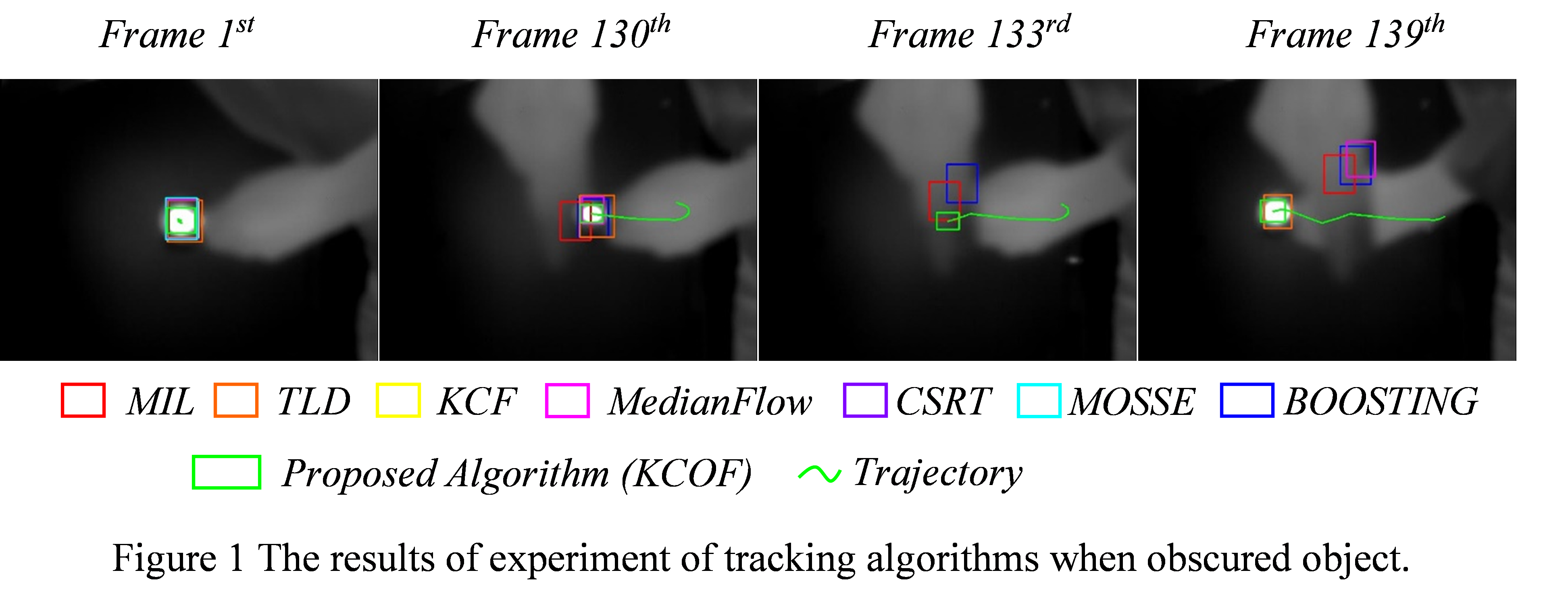The 87$^{\mathrm{th}}$, 90$^{\mathrm{th}}$, 92$^{\mathrm{nd}}$, and 96$^{\mathrm{th}}$ frames show the object moving from right to left, while thermal noise moves from left to right with greater intensity and intersects with the tracked object. Most algorithms are tricked by thermal noise, except for the BOOSTING algorithm and the proposed algorithm, which still follow the object.

The 127$^{\mathrm{th}}$, 131$^{\mathrm{st}}$, 133$^{\mathrm{rd}}$, and 136$^{\mathrm{th}}$ frames represent the temporarily obscured object. Only the proposed algorithm is still tracking the right object because the BOOSTING algorithm failed.

The new proposed combination algorithm has high stability. It can track objects with variable size, fast movement, quick change of direction, and transient occlusion and may eliminate active thermal noise.

$\textbf{Evaluation Methodology.}$

This study evaluated and compared the tracking results of the tracking algorithms based on two criteria: success rate and location accuracy.

The third circumstance - there is an active thermal noise:

##### Fig. 5. Results of the experiment of tracking algorithms when having active thermal noise.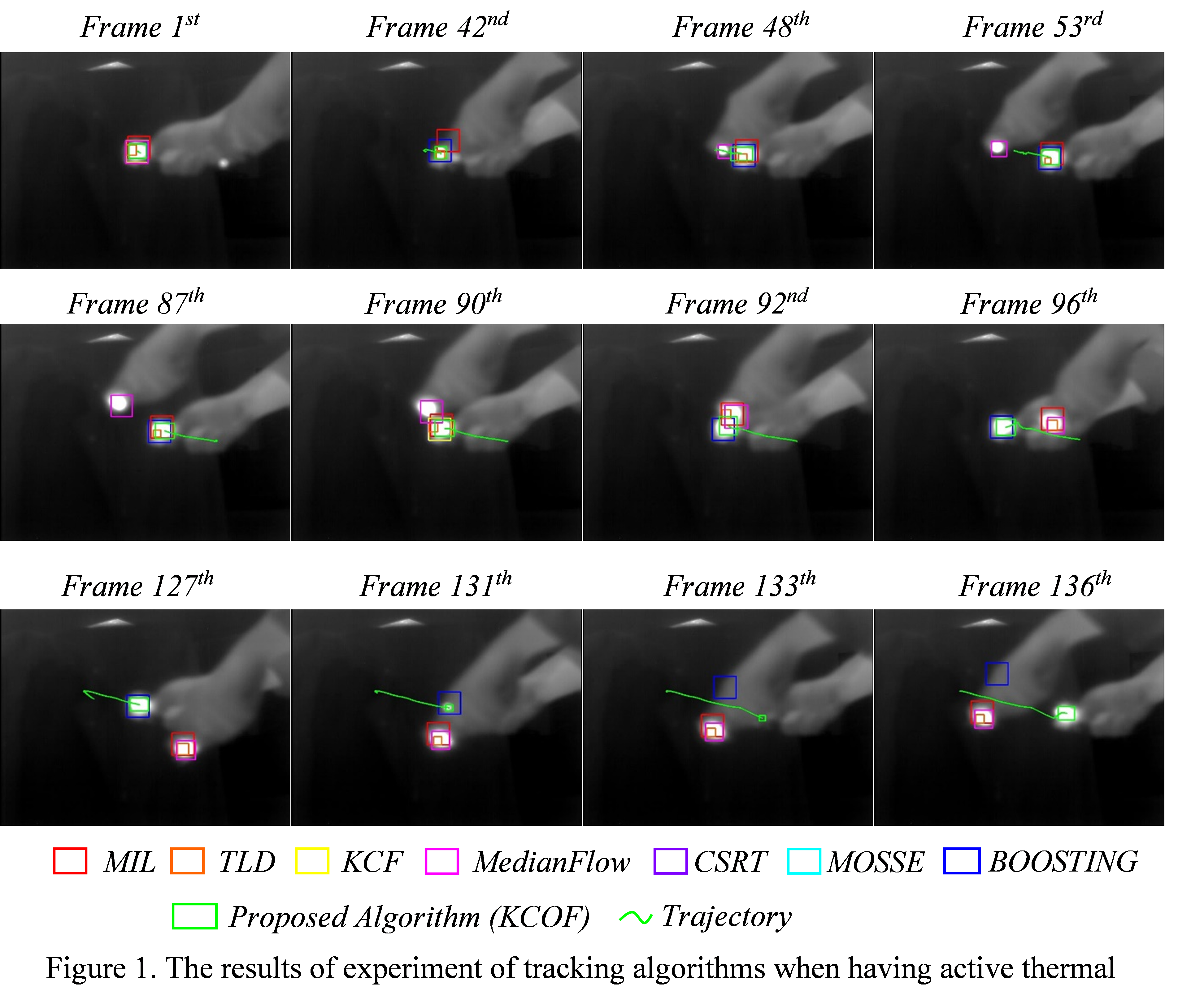The success rate was calculated based on the Jaccard index that measures similarity between two bounding boxes on the image. The ground-true bounding box is $\textit{b}$$_{t}, and the bounding box determined by the tracking algorithm is \textit{b}$$_{a}$. The Jaccard similarity index is $J=\frac{\left| b_{t}\cap b_{a}\right| }{\left| b_{t}\cup b_{a}\right| }$, where $\cap$ and $\cup$ are, respectively, the intersection and union operators of two regions determined by bounding boxes $\textit{b}$$_{t} and \textit{b}$$_{a}$. Where $\left| \cdot \right|$ is an operator that presents the number of pixels counted in the bounding box. The number of frames with a Jaccard value greater than a threshold [0,1] to the total number of frames was determined to assess the performance of the tracking algorithm with multiple thermal imaging sequences. Fig. 6 shows the graphs of this rate according to the threshold on the left.

The location accuracy of the tracking algorithm is determined based on calculating the distance between the center of the ground-true bounding box and the center-bounding box found by the tracking algorithm. Accordingly, to determine the average accuracy of multiple video sequences, the rate of the number of frames with an accuracy greater than a position error threshold to the total number of frames was calculated. Fig. 6 presents graphs of this rate according to the position error threshold on the right.

##### Fig. 6. Success rate and position accuracy of tracking algorithms.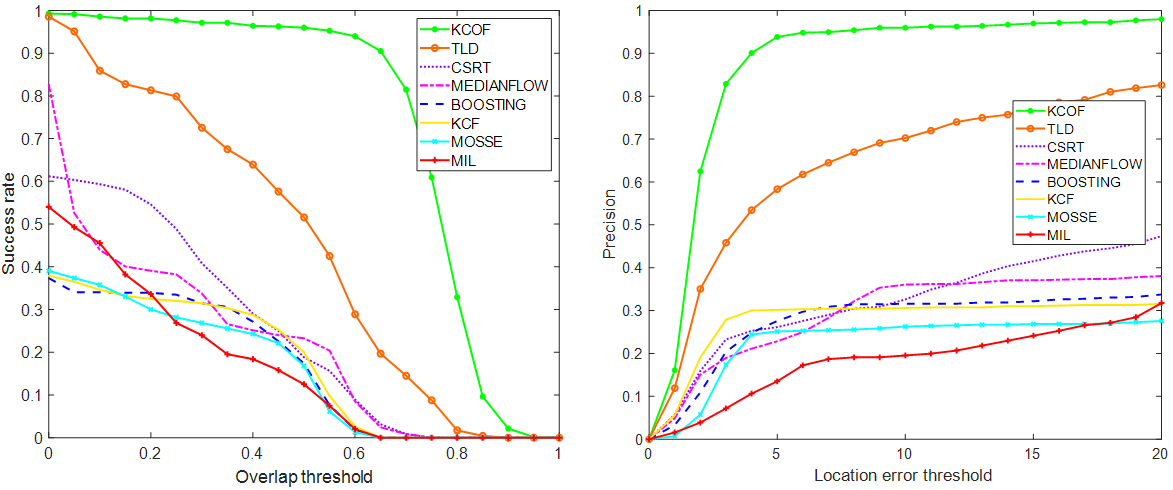The area under the curve (AUC) was used to compare the results between the algorithms according to the graphs in Fig. 6. The results are shown in Table 1 with the normalized values to [0,1].

Fig. 6 and Table 1 show that the proposed new algorithm (KCOF) has an outstanding object tracking rate and position accuracy compared to the other typical algorithms.

The proposed algorithm on a Core i7-6820HQ PC and an embedded device MPSoC UltraScale+ ZU4EV-1E had an approximate processing speed of 110 FPS and 80 FPS, respectively. Therefore, the new proposed algorithm can be performed in real-time applications under the condition of limited computational resources.

##### Table 1. The AUC values of the graphs.
 Algorithms Success rate Precision Proposed Algorithm (KCOF) 0.746 0.872 TLD 0.452 0.637 CSRT 0.245 0.320 MEDIANFLOW 0.210 0.296 BOOSTING 0.163 0.275 KCF 0.168 0.280 MOSSE 0.154 0.229 MIL 0.160 0.182

## 5. Conclusion

This paper presented a new algorithm used for tracking systems of moving objects in the thermal image. The proposed algorithm combines the advantages of object tracking algorithms, such as Camshift, Kalman, and Optical Flow, to deal with many complex circumstances of thermal objects in a video.

The experiments proved that the efficiency of the new algorithm is better than the existing separate algorithms. It has met the real-time requirement and eliminated all negative factors affecting the ability of object tracking systems, such as resizing objects, moving and quickly changing direction, being transient occlusion, or active thermal noises. Compared to other modern algorithms, the new proposed algorithm has higher stability. Therefore, it can be applied in real-time demanding problems with limited calculation resources.

### REFERENCES

1
Balaji S., Karthikeyan S., A survey on moving object tracking using image processing, in 2017 11th international conference on intelligent systems and control (ISCO). 2017. IEEE.2
Liu S., Liu Z., 2017, Multi-channel CNN-based object detection for enhanced situation awareness, arXiv preprint arXiv:1712.000753
Withey M., 2010., Infrared countermeasure flares, The Imaging Science Journal, Vol. 58, No. 5, pp. 295-2994
Chu H., et al., 2007, Object tracking algorithm based on camshift algorithm combinating with difference in frame, in 2007 IEEE International Conference on Automation and Logistics. IEEE.5
Xu T., Zhu X.-p., Zhang X.-f., 2011., Infrared imaging Maneuvering Reentry Vehicle counter target lost algorithm using Modified Gain Extended Kalman Filter, in 2011 International Conference on Electronics, Communications and Control (ICECC). IEEE.6
Thome S., Scherer-Negenborn N., Arens M., 2018, Comparing visual tracker fusion on thermal image sequences, in 2018 21st International Conference on Information Fusion (FUSION). IEEE.7
Farnebäck G., 2003, Two-frame motion estimation based on polynomial expansion, in Scandinavian conference on Image analysis. Springer8
Sharmin N., Brad R., 2012., Optimal filter estimation for Lucas-Kanade optical flow, Sensors, Vol. 12, No. 9, pp. 12694-127099
Salhi A., Jammoussi A.Y., 2012., Object tracking system using Camshift, Meanshift and Kalman filter, World Academy of Science Engineering and Technology, Vol. 64, pp. 674-67910
Swalaganata G., Affriyenni Y., 2018, Moving object tracking using hybrid method, in 2018 International Conference on Information and Communications Technology (ICOIACT). IEEE.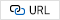11
Pérez J.S., Meinhardt-Llopis E., Facciolo G., 2013, TV-L1 optical flow estimation, Image Processing On Line 2013, pp. 137-15012
Nguen N., Hoa T.V., Vi T.N., 2021., Implementing non-uniformity correction algorithm for infrared focal plane array based on MPSoC, National Association of Scientists, Vol. 1, No. 66, pp. 14-2113
Zhang K., Song H., 2013, Real-time visual tracking via online weighted multiple instance learning, Pattern recognition, Vol. 46, No. 1, pp. 397-41114
Kalal Z., Mikolajczyk K., Matas J., 2012, Tracking-learning-detection, IEEE transactions on pattern analysis and machine intelligence, Vol. 34, No. 7, pp. 1409-142215
Henriques J.F., et al. , 2014., High-speed tracking with kernelized correlation filters, IEEE transactions on pattern analysis and machine intelligence, Vol. 37, No. 3, pp. 583-59616
Varfolomieiev A., Lysenko O., 2016., An improved algorithm of median flow for visual object tracking and its implementation on ARM platform, Journal of Real-Time Image Processing, Vol. 11, No. 3, pp. 527-53417
Lukezic A., et al. , 2017, Discriminative correlation filter with channel and spatial reliability, in Proceedings of the IEEE conference on computer vision and pattern recognition18
Danelljan M., et al. , 2014, Accurate scale estimation for robust visual tracking, in British Machine Vision Conference, Nottingham, September 1-5, 2014. Bmva Press.19
Grabner H., Grabner M., Bischof H., 2006, Real-time tracking via on-line boosting, in Bmvc. Citeseer## Author

Nguyen Ngoc Hung received his B.E. degree in Control System Designing, M.E. degree in Control and Automation Engineering from Le Quy Don Technical University (LQDTU), Vietnam, in 2010 and 2014, respectively. He has been working as a lecturer at Department of Aerospace Control Systems, LQDTU since 2011. His research interests include Image Processing for thermal cameras, Digital Signal Processing, and Intelligent Controller Designing.

Cao Huu Tinh received his Ph.D. degree in Control Engineering and Automation from Le Quy Don Technical University, Vietnam, in 2015. His main research interest is missile guidance and control systems.

Nguyen Vi Thuan received his M.S.E degree in Control and Automation Engineering from Le Quy Don Technical University (LQDTU), Vietnam in 2013. He received a Ph.D. in Control and Automation Engi-neering from Le Quy Don Technical University in 2018. He is currently a lecturer at the Department of Aerospace Control Systems, LQDTU. His research interests include Control and Estimation Theory, Optimization; Advanced Missile Guidance, Navigation, and Control; Intelligent Control.

Pham Ngoc Van received his M.S.E degree in Control and Automation Engineering from Le Quy Don Technical University (LQDTU), Vietnam, in 2006. He is currently a lecturer at the Department of Aerospace Control Systems, LQDTU. His research interests include Control and Estimation Theory, On-board Control systems, Advanced Missile guidance, Navigation, and Control.